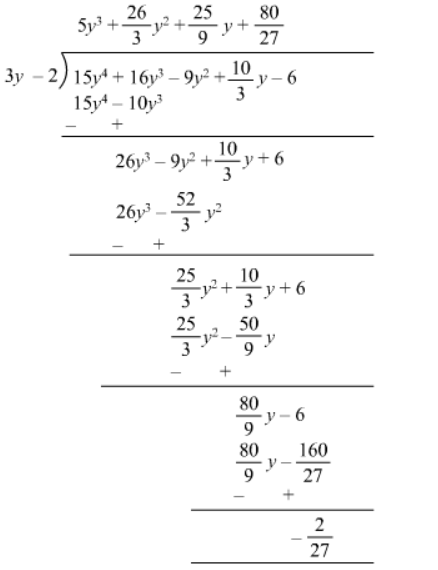# Divide the following Write down the coefficients of the terms in the quotient.`
Question:

Divide $15 y^{4}+16 y^{3}+\frac{10}{3} y-9 y^{2}-6$ by $3 y-2$. Write down the coefficients of the terms in the quotient.

Solution:$\therefore$ Quotient $=5 y^{3}+(26 / 3) y^{2}+(25 / 9) y+(80 / 27)$

Remainder $=(-2 / 27)$

Coefficient of y3 = 5

Coefficient of y2 = (26/3)

Coefficient of y = (25/9)

Constant = (80/27)